## research papersJOURNAL OFSYNCHROTRONRADIATION
ISSN: 1600-5775
Volume 26| Part 3| May 2019| Pages 756-761

## Probing the spatial coherence of wide X-ray beams with Fresnel mirrors at BL25SU of SPring-8

aDepartment of Precision Engineering, School of Engineering, The University of Tokyo, Hongo 7-3-1, Bunkyo-ku, Tokyo 113-8656, Japan, and bJapan Synchrotron Radiation Research Institute (JASRI), Kouto 1-1-1, Sayo-cho, Sayo-gun, Hyogo 679-5198, Japan
*Correspondence e-mail: mimura@edm.t.u-tokyo.ac.jp

(Received 28 June 2018; accepted 20 February 2019; online 23 April 2019)

Probing the spatial coherence of X-rays has become increasingly important when designing advanced optical systems for beamlines at synchrotron radiation sources and free-electron lasers. Double-slit experiments at various slit widths are a typical method of quantitatively measuring the spatial coherence over a wide wavelength range including the X-ray region. However, this method cannot be used for the analysis of spatial coherence when the two evaluation points are separated by a large distance of the order of millimetres owing to the extremely narrow spacing between the interference fringes. A Fresnel-mirror-based optical system can produce interference patterns by crossing two beams from two small mirrors separated in the transverse direction to the X-ray beam. The fringe spacing can be controlled via the incidence angles on the mirrors. In this study, a Fresnel-mirror-based optical system was constructed at the soft X-ray beamline (BL25SU) of SPring-8. The relationship between the coherence and size of the virtual source was quantitatively measured at 300 eV in both the vertical and horizontal directions using the beam. The results obtained indicate that this is a valuable method for the optimization of optical systems along beamlines.

### 1. Introduction

Brilliant and highly coherent X-ray beams have become available with the appearance of third-generation synchrotron radiation sources and X-ray free-electron lasers (XFELs). This has prompted the development of various X-ray imaging methods using coherent X-rays, such as phase-contrast imaging, coherent X-ray diffraction imaging and in-line holography (Snigirev et al., 1995; Miao et al., 1999; Spanne et al., 1999). An X-ray nanofocusing system with diffraction-limited performance has also been constructed (Mimura et al., 2010).

The design and construction of optical systems for carrying out such techniques requires specific beam characteristics in terms of not only brightness but also coherence, which is classified into spatial coherence and temporal coherence. A synchrotron radiation source can provide highly monochromatic X-rays by using a high-performance monochromator. The temporal coherence of an X-ray beam can be controlled to be sufficiently high so as to have no effect on imaging applications and focusing performances. However, the spatial coherence at the apparatus for these applications depends on the selected energy of the X-rays and the parameters of the optical system, such as the geometrical arrangement and slit width.

One purpose of the coherence measurement in this study is to aid in the design of a new soft X-ray nanofocusing system that uses an ellipsoidal mirror at the soft X-ray beamline (BL25SU) of SPring-8 (Motoyama et al., 2016; Mimura et al., 2018). The coherence property of the incident beam has an influence on the intensity, size and coherence width of the focusing beam. At BL25SU of SPring-8, the beam sizes of the soft X-ray beams are of the order of millimetres.

Spatial coherence has been probed using various methods in X-ray domains (Ishikawa, 1988; Trebes et al., 1992; Marconi et al., 1997; Kohn et al., 2000; Lin et al., 2003; Suzuki, 2004; Pfeiffer et al., 2005; Snigirev et al., 2009) and has been recently investigated by analysis of the single-shot diffraction patterns generated by inserting defined objects such as double pinholes and gold colloidal particles into the focusing beams (Vartanyants et al., 2011; Gutt et al., 2012; Inoue et al., 2015; Kobayashi et al., 2018). However, it becomes difficult to apply these methods to the determination of transverse coherence when the two evaluation points are separated by a large distance of the order of millimetres due to the narrower spacing of the interference fringe as described below.

The degree of coherence between two incoming beams has been measured on the basis of the visibility of the interference profile (Takayama et al., 1998; Paterson et al., 2001). For such analysis, the interference pattern should be sufficiently wide to be analysed with an imaging detector. For example, when the wavelength (λ) of the X-rays is 1 nm, the separation (d) of the two slits is 100 µm and the distance (L) between the slits and the detector is 10 m, the peak-to-peak separation (Λ) in the fringes is calculated to be as small as 100 µm from the following equation,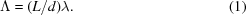Although the interval increases with increasing distance between the double slits and the detector, the intensity of the image decreases significantly.

In this study, a Fresnel-mirror-based optical system was constructed to measure the degree of spatial coherence for millimetre-size soft X-ray beams at BL25SU of SPring-8. In a Fresnel-mirror-based optical system, two grazing incidence mirrors are arranged so that the two beams reflecting at each mirror generate interference patterns, as shown in Fig. 1. This optical setup is suitable for the coherence evaluation of the wide X-ray beam, because one can increase the spacing of the interference fringe by controlling the difference between the deflection angles at the two mirrors. This method was previously utilized to measure the spatial coherence of a hard X-ray beam at a synchrotron radiation facility and to measure that of an extreme ultraviolet beam in a high-order harmonic generation system (Fezzaa et al., 1997; Le Déroff et al., 2000). Although an interferometer system that uses four mirrors to measure the coherence property of wide X-ray beams has been proposed, the optical system is rather complicated (Cash et al., 2000).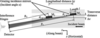Figure 1 Schematic of the Fresnel-mirror-based optical system for the evaluation of the spatial coherence of X-ray beams.

We will describe the evaluation of the degree of spatial coherence of the soft X-ray beam at 300 eV with a transverse distance of more than 1 mm. The width of the slit placed 18 m upstream of the double mirrors was varied to change the spatial coherence at the mirrors. There is a marked difference in the spatial coherence properties between the horizontal and vertical directions owing to the characteristics of the source under SPring-8 specifications. This difference can be observed by comparing the results obtained before and after rotating the entire Fresnel-mirror-based optical system by 90°.

### 2. Theoretical model and analytical procedure

In interferometry with Fresnel mirrors, two parts of an incident X-ray beam are reflected at two small mirrors under grazing incidences. An interference fringe pattern is produced because of the overlapping of these reflected beams. The acceptances of the two mirrors are sufficiently small relative to the size of the incident X-ray beam. When the detector is placed in the far field of these mirrors, the degree of coherence analysed from the visibility of the interference fringe can be regarded as the degree of coherence between two X-rays reflecting at the central points of the two mirrors.

The aperture width w of each mirror is equal to lsinα, where l is the longitudinal length of the mirror and α is the incidence angle. Flat or cylindrical shapes of the wavefront at the detector are preferable from the viewpoint of the analysis. In the Fraunhofer diffraction equation, the wavefront inside the central band of a propagating beam is assumed to be cylindrical, although w2/8Rλ must be satisfied, where λ is the wavelength and R is the distance between the mirror and the detector. The beam reflected at the mirror travels while expanding in the horizontal direction as a result of the diffraction effect, as shown in Fig. 1.

Here, we develop an expression for the intensity and phase profiles of the beam from the upstream mirror (M1) in Fig. 2. The (x, y, z) reference frame is defined by the beam propagation direction (z), the vertical direction (y) and the horizontal direction (x), with +x pointing towards the storage ring. On an arbitrary horizontal line at the detector represented by y = y0, the observed intensity distribution I1(x, y0) is given by the approximate formula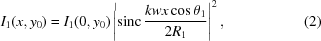where I1(0, y0) is the maximum intensity on the arbitrary horizontal line, k is the wavenumber, R1 is the distance between M1 and the detector, and θ1 is the angle between the reflected beam and the z axis. As the diffraction pattern is given by a rectangular aperture, the intensity profile in x is expressed by the square of a sinc function, whereas the width of the central band is 2λR1/(wcosθ1). Inside this region, the phase profile is linear with x and is given by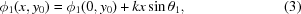where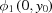is the phase of the propagating beam at the central point on the arbitrary horizontal line.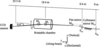Figure 2 Layout of the Fresnel-mirror-based optical system at the soft X-ray beamline of SPring-8.

In this experiment, the positions and inclinations of the two mirrors are adjusted so that the central bands of the intensity profiles of the two beams overlap at the detector. If the relationship between the two beams is perfectly coherent, then an interference fringe pattern can be clearly observed.

The intensity profile of an interference fringe pattern is calculated by summing the complex wavefields and squaring the absolute amplitude of the total wavefield. Here, I3 is the intensity profile observed at the detector when the two beams overlap. I1 and I2 are the intensity profiles of the single beams from the upstream mirror M1 and the downstream mirror M2, respectively. The subscripts of θ1, θ2, ϕ1 and ϕ2 indicate the mirror number. On the basis of the above definitions, I3 is given by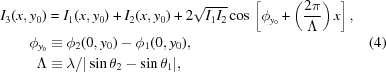where ϕy0 is a constant and Λ is the interval of the fringe. If the relationship between the two beams is characterized by partial coherence, then their complex degree of coherence is defined quantitatively by the mutual coherence function. The intensity profile I3 is updated to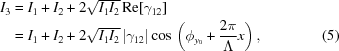where γ12 is the complex degree of coherence. The absolute value of γ12 is defined as the degree of coherence, which takes values between 0 and 1. The degree of coherence can be analysed from the normalization of the interference intensity profile. The normalized profile is represented by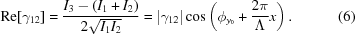For precise analysis, |γ12| is determined by the amplitude of the sine curve fit to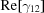.

### 3. Optical system setup of Fresnel mirrors

A Fresnel-mirror-based optical system was constructed at the soft X-ray beamline (BL25SU) of SPring-8, where a twin helical undulator has been installed. Measurement experiments of the transverse coherence were conducted at the BL25SU-a beamline. Soft X-rays are monochromated with an optical system that consists of three mirrors and a varied-line-spacing plane grating (Senba et al., 2016). Soft X-rays are focused on a transversal plane in the vertical direction, on which the S2a slit is placed and dispersed so that each wavelength satisfies the diffraction grating equation. The mean energy and energy bandwidth of the X-rays are determined by the slit position and opening width, respectively. The soft X-ray beam after the S2a slit propagates with a vertical divergence angle of at least the order of 100 µrad, and horizontally diverges with an angle of the order of 10 µrad. To suppress the large beam divergence in the vertical direction, a collimator mirror (MCa) was set 270 mm downstream of S2a. The SCa slit, which is composed of four blades, was installed 0.4 m downstream from S2a as the virtual source for this study. The beam energy and energy bandwidth were set to 300 eV and E/ΔE = 10 000, respectively. The sizes of the beam are 1.27 mm and 0.45 mm in the horizontal and vertical directions, respectively, when the X-ray beam impinges on the SCa slit.

The Fresnel-mirror-based optical system shown in Fig. 2was constructed 18 m downstream of SCa. The diameter of the two plane mirrors was 25.4 mm and the incidence angle was approximately 0.2°. A CCD detector (PIXIS-XO:2048B, Princeton Instruments) with a pixel size of 13.5 µm was located 4.9 m downstream of the Fresnel mirrors. The detector can move horizontally and vertically to track the beam positions during the alignment of the two mirrors.

Fig. 3shows the two mirrors held on a five-axis stage to adjust their inclinations and positions. The incident angles corresponding to the pitching angles determine the intervals between the interference fringes. The rolling angles are tuned so that the fringes are parallel to the y direction and the effect of the vertical coherence property on the horizontal measurement is eliminated. The distance between the central points of two mirrors in the z direction can be changed by moving the downstream mirror with a one-axis motor stage. The distance Δx in Fig. 3is the transverse distance between the two mirrors, which is adjusted by the x stages of the mirrors. The whole system shown in Fig. 3can be rotated by 90° around the optical axis. Owing to geometric constraints, the settable range of Δx is expressed as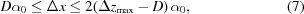where D and α0 are the mirror length and incident angle, respectively, and Δzmax is the maximum distance between the two mirrors in the z direction. For example, for the settings α0 = 0.2° and Δzmax = 211 mm, the measurable values of Δx range from 88 µm to 1296 µm.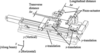Figure 3 Installed alignment system for two small mirrors in the Fresnel-mirror-based optical system.

Table 1shows the geometric parameters of the two mirrors. The slit size of SCa is varied under these geometrical conditions. The relationships between the slit width and the degree of coherence for the two beams reflected at the two mirrors were investigated in the horizontal and vertical directions. Δx and Δy were also varied.

 Table 1Experimental conditions for measuring the degree of spatial coherence using a Fresnel-mirror-based optical system
Direction Transverse distance Δx or Δy (µm) Longitudinal distance Δz (mm) Interval between interference fringes Λ (µm) Difference between deflection angles (µrad)
Horizontal 171 51 142 29.1
Horizontal 1232 199 170 24.3
Vertical 224 51 180 23.0
Vertical 1273 199 136 30.4

Low temporal coherence deteriorates the visibility of an interference fringe pattern when there is a difference in the optical path length between the two beams. A difference of the order of 10 µm has a significant impact on the visibility at a wavelength of 4.13 nm with E/ΔE = 10 000. Under the geometrical conditions shown in Table 1, the maximum difference in the optical path is 4 µm; thus, the effect of temporal coherence is negligible.

### 4. Measurements of coherence properties

Fig. 4shows representative intensity distributions and their cross-sectional profiles recorded by the CCD camera during the measurement of the degree of coherence in the horizontal direction, where the width of the SCa slit is 10 µm and the transverse distance between the two mirrors is 171 µm. The line with circles in Fig. 4(d) shows the intensity profile measured when only the upstream mirror M1 reflected a beam, which is the square of the sinc function profile corresponding to the Fraunhofer diffraction pattern of X-rays propagating from a small rectangular aperture. Interference fringes appeared on the superposition of the two beams from the two mirrors. The spacing of the interference fringe was optimized by tuning the deflection angle of M2 for the observation using the CCD camera. The cross-sectional profiles were normalized using equation (6) and were fitted by a sine curve profile as shown in Fig. 5. This fitting calculation was performed within two periods of the interference fringe pattern within the central bands. The degree of spatial coherence was determined by the amplitude of the fitted sine curve. To increase the accuracy, five fitted curves obtained at different lines were used and their amplitudes were averaged. Fig. 6shows the interference images when Δx is 171 µm and the width of the SCa slit is changed from 10 µm to the full opening of 20 000 µm, which indicates that the fringe visibility decreases with increasing slit width.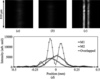Figure 4 Intensity images and cross-sectional profiles of a measured interference fringe pattern. The X-ray energy is 300 eV. Intensity images of (a) the beam from M1, (b) the beam from M2 and (c) the overlapped beams. (d) Cross-sectional profiles in the horizontal direction.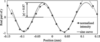Figure 5 Determination of the degree of coherence. A sine curve is used to fit the normalized intensity profile of the interference fringe shown in Fig. 4.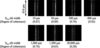Figure 6 Comparison between the degrees of coherence for different SCa slit widths as a virtual source and measured intensity images of the interference fringe of the X-ray beams.

In this study, the width of the SCa slit and Δx or Δy were varied as parameters to clarify the spatial coherence properties of wide soft X-ray beams. Fig. 7(a) shows a graph of the relationship between the horizontal slit width and the degree of spatial coherence when Δx is 171 µm and 1232 µm. The degree of coherence monotonically increases with decreasing slit width for both values of Δx. It was thus confirmed that the degree of spatial coherence is improved by closing the SCa slit.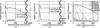Figure 7 Relationship between the degree of coherence and the SCa slit width in the (a) horizontal and (b) vertical directions. Δx is the horizontal distance between the central points of the two mirrors and Δy is the vertical distance. These results were measured at an X-ray energy of 300 eV. (c) Degree of coherence calculated on the basis of the van Cittert–Zernike's theorem; Δh is the transverse distance between the central points of the mirrors.

The coherence properties were also investigated in the vertical direction under almost the same experimental conditions as those for the horizontal direction. The optical system was rotated by 90° and aligned to observe the interference pattern using the CCD camera. Fig. 7(b) shows the coherence properties obtained by varying the vertical width of the SCa slit, which clearly indicates that the coherence properties in the vertical direction are superior to those in the horizontal direction.

The degree of coherence was calculated on the basis of van Cittert–Zernike's theorem, as shown in Fig. 7(c), in which the soft X-ray beam was assumed to be incoherent light at the SCa slit. The measured degree of coherence is higher than the calculated values in both the horizontal and vertical directions, which indicates that the soft X-rays at the SCa slit have a partial coherence property.

### 5. Discussion and summary

The degree of spatial coherence of the soft X-ray beam at BL25SU-a of SPring-8 was measured using a Fresnel-mirror-based optical system. The effect of the upstream optics on the spatial coherence on the downstream side, where the soft X-ray beam has diverged, could be quantitatively evaluated. The degree of spatial coherence in both the horizontal and vertical directions tends to decrease upon increasing width of the slit placed far upstream of the Fresnel mirrors. The degree of spatial coherence was higher in the vertical direction than in the horizontal direction for the X-ray source properties of the synchrotron radiation source.

According to the experimental results, when the slit widths are approximately 10 µm and 20 µm in the horizontal and vertical directions, respectively, the soft X-ray beam is fully coherent in a square area of the beam of 1.2 mm2 at the Fresnel mirrors. Thus, the diffraction-limited soft X-ray focusing is theoretically possible under these conditions.

The results indicate that the Fresnel-mirror-based system can be used to measure the spatial coherence properties of wide X-ray beams under highly monochromatic conditions. This optical system can also be used to determine the degree of coherence of X-ray pulses for XFELs owing to the possibility of single-shot measurements. Precise quantitative information on coherence will lead to further improvements of soft X-ray beamlines and various methods applying coherence at synchrotron radiation and XFEL facilities.

### Funding information

The synchrotron radiation experiments were performed at the BL25SU-a of SPring-8 with the approval of the Japan Synchrotron Radiation Research Institute (JASRI) (Proposal No. 2015 A1148). This work was supported by a Grant-in-Aid for Scientific Research (A) from the Ministry of Education, Sports, Culture, Science and Technology, Japan (MEXT).

### ReferencesJOURNAL OFSYNCHROTRONRADIATION